Within Teamwork, the progress is recorded individually for each task, meaning the progress of subtasks does not affect the progress of the parent task at all.

The formula of calculation is `∑d*p / ∑d`, where "d" is task duration in days, "p" is the task progress and "∑" stands for a sum of all loaded tasks.

This will go through all active tasks (visible on screen), add up duration in days multiplied by progress, and divide it by the sum of durations in days for all tasks.

Note: Completed tasks are not loaded by default. Unless you click Show Completed, the task list progress will be represented based on the loaded tasks instead of the whole task list.

For example, if you have a task list with two tasks with one task dependent on a single subtask

• Task 1: "Get DeLorean" has a duration of 12 days - 63% of the total task list percentage if completed.
• Task 2: "Reach 88 mph" has a duration of 7 days - 37% of the task list progress if completed.
• Subtask: "Update engine" has a duration of 5 days - Progress for the subtask will not count towards the task list progress on the Gantt chart.
Task list on creation: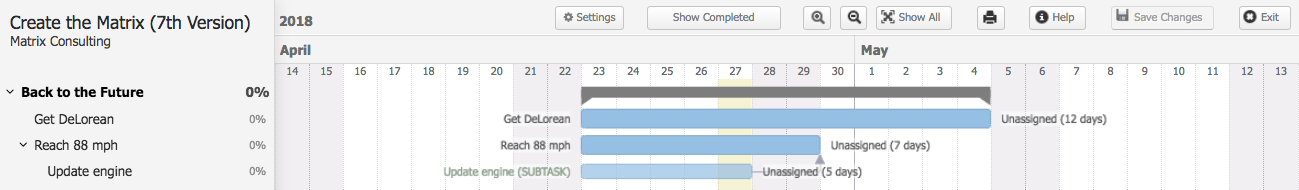If you mark the progress on:

• "Get DeLorean" to 30% complete
• "Reach 88 mph" to 70% complete
• "Update engine" left at 0% complete
• The total progress for the task list is 35% complete:
• `∑d*p / ∑d = ∑(12*30),(7*70),(5*0) / ∑(12,7,5) = 850 / 24 = 35%`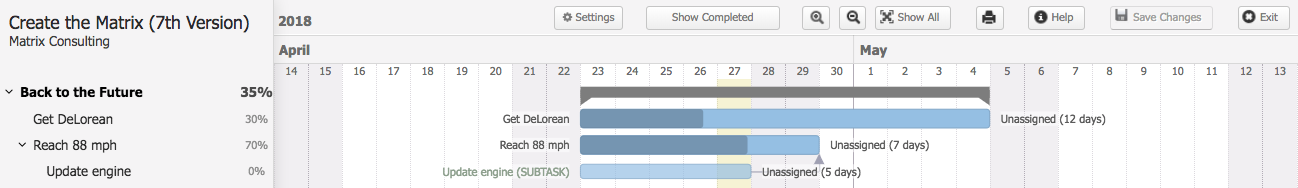If you complete "Update engine," this will mean that the task list is 56% complete:

• `∑d*p / ∑d = ∑(12*30),(7*70),(5*100) / ∑(12,7,5) = 1350 / 24 = 56.25%`
The task can be completed via the Gantt chart by clicking on the check mark on the left pane after you hover over the task: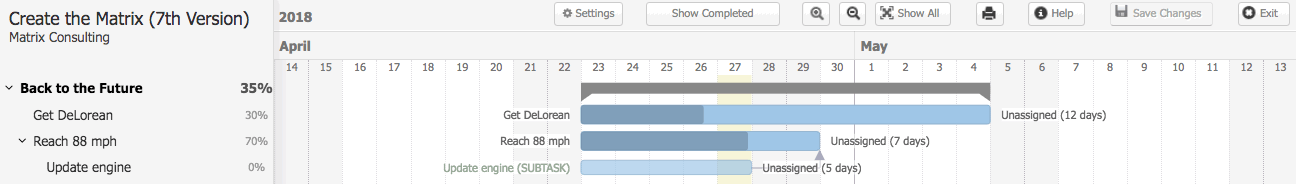Completing "Reach 88 mph" then will mean that the task list is 65% complete:

• ```∑d*p / ∑d =  ∑(12*30),(7*100),(5*100) / ∑(12,7,5) = 1560 / 24 = 65%```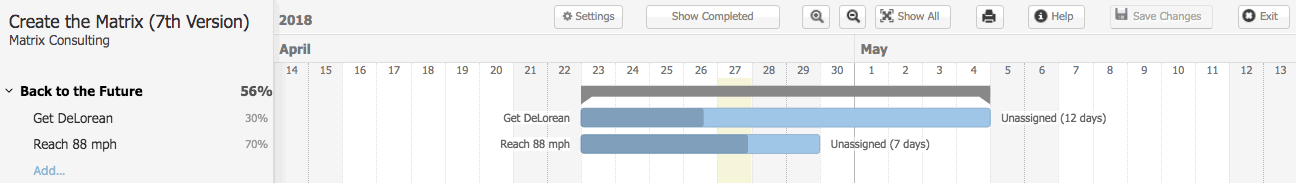When you access the Gantt chart at a later stage, the completed tasks will not appear so the current progress of the active task(s) will be what is taken for the task list progress.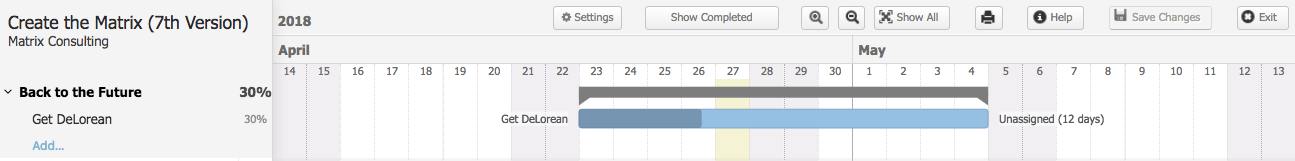You can display the completed tasks on the Gantt chart by clicking the Show Completed button at the top of the Gantt view.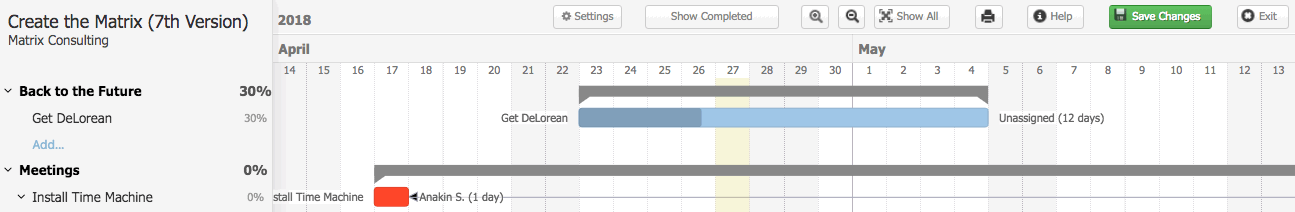The part percentage of progress that represents the 100% progress of the completed task "Reach 88 mph" will be added to the task list total, including its subtask "Update engine."

This brings you back to the following calculation:

• `∑d*p / ∑d = ∑(12*30),(7*100),(5*100) / ∑(12,7,5) = 1560 / 24 = 65%`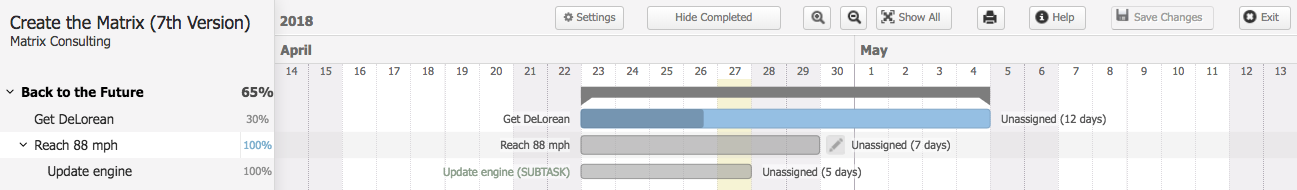For more information, see: Viewing Your Project in the Gantt Chart